# imufilter

Orientation from accelerometer and gyroscope readings

Since R2018b

## Description

The `imufilter` System object™ fuses accelerometer and gyroscope sensor data to estimate device orientation.

To estimate device orientation:

1. Create the `imufilter` object and set its properties.

2. Call the object with arguments, as if it were a function.

## Creation

### Syntax

``FUSE = imufilter``
``FUSE = imufilter('ReferenceFrame',RF)``
``FUSE = imufilter(___,Name,Value)``

### Description

example

````FUSE = imufilter` returns an indirect Kalman filter System object, `FUSE`, for fusion of accelerometer and gyroscope data to estimate device orientation. The filter uses a nine-element state vector to track error in the orientation estimate, the gyroscope bias estimate, and the linear acceleration estimate.```
````FUSE = imufilter('ReferenceFrame',RF)` returns an `imufilter` filter System object that fuses accelerometer and gyroscope data to estimate device orientation relative to the reference frame `RF`. Specify `RF` as `'NED'` (North-East-Down) or `'ENU'` (East-North-Up). The default value is `'NED'`.```

example

````FUSE = imufilter(___,Name,Value)` sets each property `Name` to the specified `Value`. Unspecified properties have default values.Example: `FUSE = imufilter('SampleRate',200,'GyroscopeNoise',1e-6)` creates a System object, `FUSE`, with a 200 Hz sample rate and gyroscope noise set to 1e-6 radians per second squared.```

## Properties

expand all

Unless otherwise indicated, properties are nontunable, which means you cannot change their values after calling the object. Objects lock when you call them, and the `release` function unlocks them.

If a property is tunable, you can change its value at any time.

Sample rate of the input sensor data in Hz, specified as a positive finite scalar.

Tunable: No

Data Types: `single` | `double` | `uint8` | `uint16` | `uint32` | `uint64` | `int8` | `int16` | `int32` | `int64`

Decimation factor by which to reduce the sample rate of the input sensor data, specified as a positive integer scalar.

The number of rows of the inputs, `accelReadings` and `gyroReadings`, must be a multiple of the decimation factor.

Tunable: No

Data Types: `single` | `double` | `uint8` | `uint16` | `uint32` | `uint64` | `int8` | `int16` | `int32` | `int64`

Variance of accelerometer signal noise in (m/s2)2, specified as a positive real scalar.

Tunable: Yes

Data Types: `single` | `double` | `uint8` | `uint16` | `uint32` | `uint64` | `int8` | `int16` | `int32` | `int64`

Variance of gyroscope signal noise in (rad/s)2, specified as a positive real scalar.

Tunable: Yes

Data Types: `single` | `double` | `uint8` | `uint16` | `uint32` | `uint64` | `int8` | `int16` | `int32` | `int64`

Variance of gyroscope offset drift in (rad/s)2, specified as a positive real scalar.

Tunable: Yes

Data Types: `single` | `double` | `uint8` | `uint16` | `uint32` | `uint64` | `int8` | `int16` | `int32` | `int64`

Variance of linear acceleration noise in (m/s2)2, specified as a positive real scalar. Linear acceleration is modeled as a lowpass filtered white noise process.

Tunable: Yes

Data Types: `single` | `double` | `uint8` | `uint16` | `uint32` | `uint64` | `int8` | `int16` | `int32` | `int64`

Decay factor for linear acceleration drift, specified as a scalar in the range [0,1]. If linear acceleration is changing quickly, set `LinearAccelerationDecayFactor` to a lower value. If linear acceleration changes slowly, set `LinearAccelerationDecayFactor` to a higher value. Linear acceleration drift is modeled as a lowpass-filtered white noise process.

Tunable: Yes

Data Types: `single` | `double` | `uint8` | `uint16` | `uint32` | `uint64` | `int8` | `int16` | `int32` | `int64`

Covariance matrix for process noise, specified as a 9-by-9 matrix. The default is:

``` Columns 1 through 6 0.000006092348396 0 0 0 0 0 0 0.000006092348396 0 0 0 0 0 0 0.000006092348396 0 0 0 0 0 0 0.000076154354947 0 0 0 0 0 0 0.000076154354947 0 0 0 0 0 0 0.000076154354947 0 0 0 0 0 0 0 0 0 0 0 0 0 0 0 0 0 0 Columns 7 through 9 0 0 0 0 0 0 0 0 0 0 0 0 0 0 0 0 0 0 0.009623610000000 0 0 0 0.009623610000000 0 0 0 0.009623610000000```

The initial process covariance matrix accounts for the error in the process model.

Data Types: `single` | `double` | `uint8` | `uint16` | `uint32` | `uint64` | `int8` | `int16` | `int32` | `int64`

Output orientation format, specified as `'quaternion'` or `'Rotation matrix'`. The size of the output depends on the input size, N, and the output orientation format:

• `'quaternion'` –– Output is an N-by-1 `quaternion`.

• `'Rotation matrix'` –– Output is a 3-by-3-by-N rotation matrix.

Data Types: `char` | `string`

## Usage

### Syntax

``[orientation,angularVelocity] = FUSE(accelReadings,gyroReadings)``

### Description

example

````[orientation,angularVelocity] = FUSE(accelReadings,gyroReadings)` fuses accelerometer and gyroscope readings to compute orientation and angular velocity measurements. The algorithm assumes that the device is stationary before the first call.```

### Input Arguments

expand all

Accelerometer readings in the sensor body coordinate system in m/s2, specified as an N-by-3 matrix. N is the number of samples, and the three columns of `accelReadings` represent the [x y z] measurements. Accelerometer readings are assumed to correspond to the sample rate specified by the SampleRate property.

Data Types: `single` | `double`

Gyroscope readings in the sensor body coordinate system in rad/s, specified as an N-by-3 matrix. N is the number of samples, and the three columns of `gyroReadings` represent the [x y z] measurements. Gyroscope readings are assumed to correspond to the sample rate specified by the SampleRate property.

Data Types: `single` | `double`

### Output Arguments

expand all

Orientation that can rotate quantities from a global coordinate system to a body coordinate system, returned as quaternions or an array. The size and type of `orientation` depends on whether the OrientationFormat property is set to `'quaternion'` or ```'Rotation matrix'```:

• `'quaternion'` –– The output is an M-by-1 vector of quaternions, with the same underlying data type as the inputs.

• `'Rotation matrix'` –– The output is a 3-by-3-by-M array of rotation matrices the same data type as the inputs.

The number of input samples, N, and the DecimationFactor property determine M.

You can use `orientation` in a `rotateframe` function to rotate quantities from a global coordinate system to a sensor body coordinate system.

Data Types: `quaternion` | `single` | `double`

Angular velocity with gyroscope bias removed in the sensor body coordinate system in rad/s, returned as an M-by-3 array. The number of input samples, N, and the DecimationFactor property determine M.

Data Types: `single` | `double`

## Object Functions

To use an object function, specify the System object as the first input argument. For example, to release system resources of a System object named `obj`, use this syntax:

`release(obj)`

expand all

 `tune` Tune `imufilter` parameters to reduce estimation error
 `step` Run System object algorithm `release` Release resources and allow changes to System object property values and input characteristics `reset` Reset internal states of System object

## Examples

collapse all

Load the `rpy_9axis` file, which contains recorded accelerometer, gyroscope, and magnetometer sensor data from a device oscillating in pitch (around y-axis), then yaw (around z-axis), and then roll (around x-axis). The file also contains the sample rate of the recording.

```load 'rpy_9axis.mat' sensorData Fs accelerometerReadings = sensorData.Acceleration; gyroscopeReadings = sensorData.AngularVelocity;```

Create an `imufilter` System object™ with sample rate set to the sample rate of the sensor data. Specify a decimation factor of two to reduce the computational cost of the algorithm.

```decim = 2; fuse = imufilter('SampleRate',Fs,'DecimationFactor',decim);```

Pass the accelerometer readings and gyroscope readings to the `imufilter` object, `fuse`, to output an estimate of the sensor body orientation over time. By default, the orientation is output as a vector of quaternions.

`q = fuse(accelerometerReadings,gyroscopeReadings);`

Orientation is defined by the angular displacement required to rotate a parent coordinate system to a child coordinate system. Plot the orientation in Euler angles in degrees over time.

`imufilter` fusion correctly estimates the change in orientation from an assumed north-facing initial orientation. However, the device's x-axis was pointing southward when recorded. To correctly estimate the orientation relative to the true initial orientation or relative to NED, use `ahrsfilter`.

```time = (0:decim:size(accelerometerReadings,1)-1)/Fs; plot(time,eulerd(q,'ZYX','frame')) title('Orientation Estimate') legend('Z-axis', 'Y-axis', 'X-axis') xlabel('Time (s)') ylabel('Rotation (degrees)')```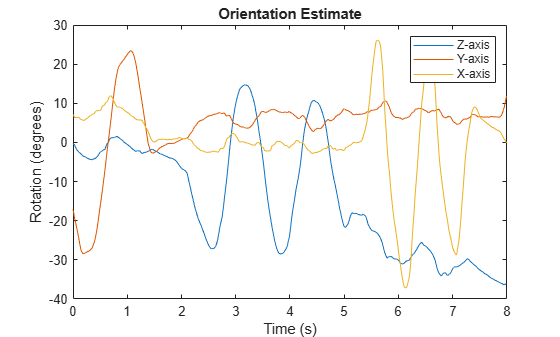Model a tilting IMU that contains an accelerometer and gyroscope using the `imuSensor` System object™. Use ideal and realistic models to compare the results of orientation tracking using the `imufilter` System object.

Load a struct describing ground-truth motion and a sample rate. The motion struct describes sequential rotations:

1. yaw: 120 degrees over two seconds

2. pitch: 60 degrees over one second

3. roll: 30 degrees over one-half second

4. roll: -30 degrees over one-half second

5. pitch: -60 degrees over one second

6. yaw: -120 degrees over two seconds

In the last stage, the motion struct combines the 1st, 2nd, and 3rd rotations into a single-axis rotation. The acceleration, angular velocity, and orientation are defined in the local NED coordinate system.

```load y120p60r30.mat motion fs accNED = motion.Acceleration; angVelNED = motion.AngularVelocity; orientationNED = motion.Orientation; numSamples = size(motion.Orientation,1); t = (0:(numSamples-1)).'/fs; ```

Create an ideal IMU sensor object and a default IMU filter object.

```IMU = imuSensor('accel-gyro','SampleRate',fs); aFilter = imufilter('SampleRate',fs); ```

In a loop:

1. Simulate IMU output by feeding the ground-truth motion to the IMU sensor object.

2. Filter the IMU output using the default IMU filter object.

```orientation = zeros(numSamples,1,'quaternion'); for i = 1:numSamples [accelBody,gyroBody] = IMU(accNED(i,:),angVelNED(i,:),orientationNED(i,:)); orientation(i) = aFilter(accelBody,gyroBody); end release(aFilter) ```

Plot the orientation over time.

```figure(1) plot(t,eulerd(orientation,'ZYX','frame')) xlabel('Time (s)') ylabel('Rotation (degrees)') title('Orientation Estimation -- Ideal IMU Data, Default IMU Filter') legend('Z-axis','Y-axis','X-axis') ```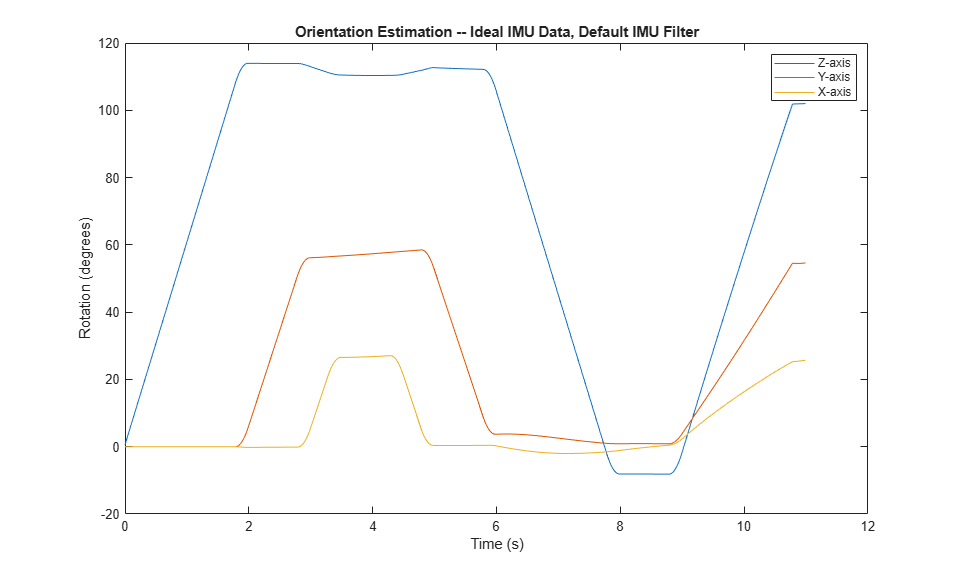Modify properties of your `imuSensor` to model real-world sensors. Run the loop again and plot the orientation estimate over time.

```IMU.Accelerometer = accelparams( ... 'MeasurementRange',19.62, ... 'Resolution',0.00059875, ... 'ConstantBias',0.4905, ... 'AxesMisalignment',2, ... 'NoiseDensity',0.003924, ... 'BiasInstability',0, ... 'TemperatureBias', [0.34335 0.34335 0.5886], ... 'TemperatureScaleFactor',0.02); IMU.Gyroscope = gyroparams( ... 'MeasurementRange',4.3633, ... 'Resolution',0.00013323, ... 'AxesMisalignment',2, ... 'NoiseDensity',8.7266e-05, ... 'TemperatureBias',0.34907, ... 'TemperatureScaleFactor',0.02, ... 'AccelerationBias',0.00017809, ... 'ConstantBias',[0.3491,0.5,0]); orientationDefault = zeros(numSamples,1,'quaternion'); for i = 1:numSamples [accelBody,gyroBody] = IMU(accNED(i,:),angVelNED(i,:),orientationNED(i,:)); orientationDefault(i) = aFilter(accelBody,gyroBody); end release(aFilter) figure(2) plot(t,eulerd(orientationDefault,'ZYX','frame')) xlabel('Time (s)') ylabel('Rotation (degrees)') title('Orientation Estimation -- Realistic IMU Data, Default IMU Filter') legend('Z-axis','Y-axis','X-axis') ```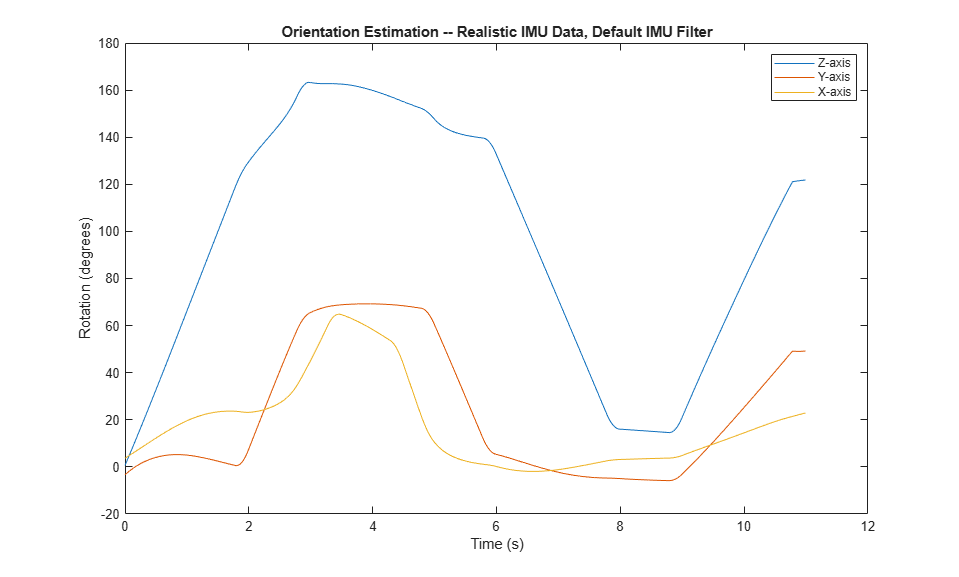The ability of the `imufilter` to track the ground-truth data is significantly reduced when modeling a realistic IMU. To improve performance, modify properties of your `imufilter` object. These values were determined empirically. Run the loop again and plot the orientation estimate over time.

```aFilter.GyroscopeNoise = 7.6154e-7; aFilter.AccelerometerNoise = 0.0015398; aFilter.GyroscopeDriftNoise = 3.0462e-12; aFilter.LinearAccelerationNoise = 0.00096236; aFilter.InitialProcessNoise = aFilter.InitialProcessNoise*10; orientationNondefault = zeros(numSamples,1,'quaternion'); for i = 1:numSamples [accelBody,gyroBody] = IMU(accNED(i,:),angVelNED(i,:),orientationNED(i,:)); orientationNondefault(i) = aFilter(accelBody,gyroBody); end release(aFilter) figure(3) plot(t,eulerd(orientationNondefault,'ZYX','frame')) xlabel('Time (s)') ylabel('Rotation (degrees)') title('Orientation Estimation -- Realistic IMU Data, Nondefault IMU Filter') legend('Z-axis','Y-axis','X-axis') ```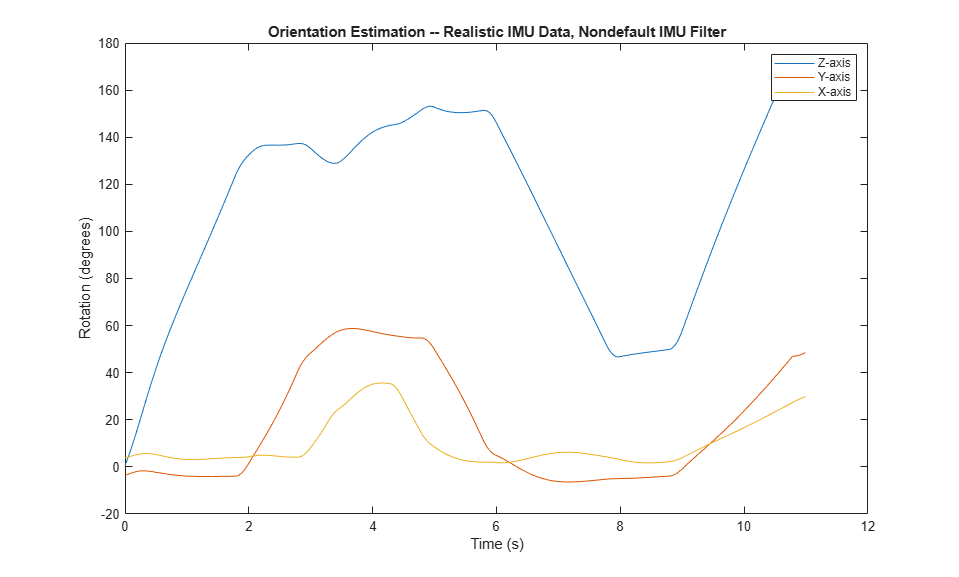To quantify the improved performance of the modified `imufilter`, plot the quaternion distance between the ground-truth motion and the orientation as returned by the `imufilter` with default and nondefault properties.

```qDistDefault = rad2deg(dist(orientationNED,orientationDefault)); qDistNondefault = rad2deg(dist(orientationNED,orientationNondefault)); figure(4) plot(t,[qDistDefault,qDistNondefault]) title('Quaternion Distance from True Orientation') legend('Realistic IMU Data, Default IMU Filter', ... 'Realistic IMU Data, Nondefault IMU Filter') xlabel('Time (s)') ylabel('Quaternion Distance (degrees)') ```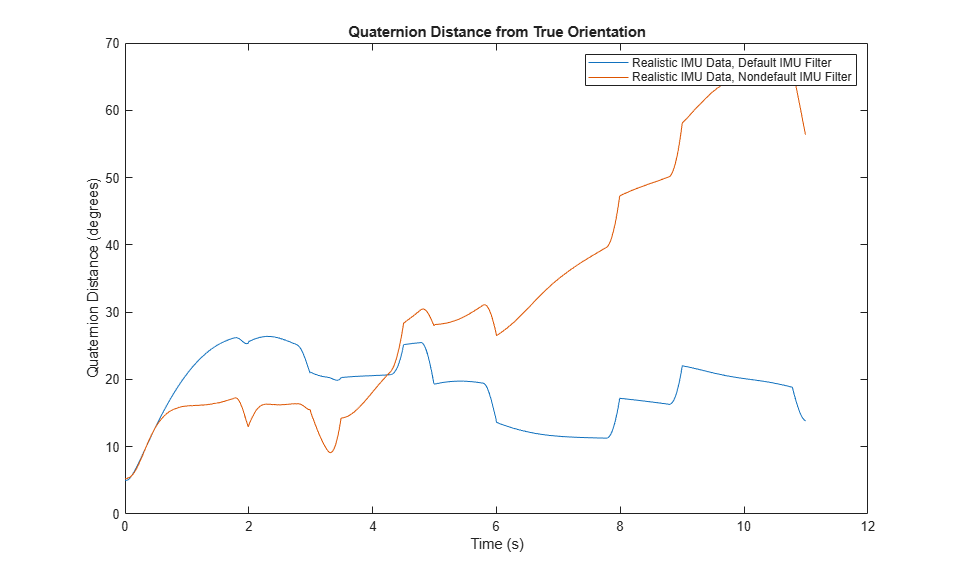This example shows how to remove gyroscope bias from an IMU using `imufilter`.

Use `kinematicTrajectory` to create a trajectory with two parts. The first part has a constant angular velocity about the y- and z-axes. The second part has a varying angular velocity in all three axes.

```duration = 60*8; fs = 20; numSamples = duration * fs; rng('default') % Seed the RNG to reproduce noisy sensor measurements. initialAngVel = [0,0.5,0.25]; finalAngVel = [-0.2,0.6,0.5]; constantAngVel = repmat(initialAngVel,floor(numSamples/2),1); varyingAngVel = [linspace(initialAngVel(1), finalAngVel(1), ceil(numSamples/2)).', ... linspace(initialAngVel(2), finalAngVel(2), ceil(numSamples/2)).', ... linspace(initialAngVel(3), finalAngVel(3), ceil(numSamples/2)).']; angVelBody = [constantAngVel; varyingAngVel]; accBody = zeros(numSamples,3); traj = kinematicTrajectory('SampleRate',fs); [~,qNED,~,accNED,angVelNED] = traj(accBody,angVelBody);```

Create an `imuSensor` System object™, `IMU`, with a nonideal gyroscope. Call `IMU` with the ground-truth acceleration, angular velocity, and orientation.

```IMU = imuSensor('accel-gyro', ... 'Gyroscope',gyroparams('RandomWalk',0.003,'ConstantBias',0.3), ... 'SampleRate',fs); [accelReadings, gyroReadingsBody] = IMU(accNED,angVelNED,qNED);```

Create an `imufilter` System object, `fuse`. Call `fuse` with the modeled accelerometer readings and gyroscope readings.

```fuse = imufilter('SampleRate',fs, 'GyroscopeDriftNoise', 1e-6); [~,angVelBodyRecovered] = fuse(accelReadings,gyroReadingsBody);```

Plot the ground-truth angular velocity, the gyroscope readings, and the recovered angular velocity for each axis.

The angular velocity returned from the `imufilter` compensates for the effect of the gyroscope bias over time and converges to the true angular velocity.

```time = (0:numSamples-1)'/fs; figure(1) plot(time,angVelBody(:,1), ... time,gyroReadingsBody(:,1), ... time,angVelBodyRecovered(:,1)) title('X-axis') legend('True Angular Velocity', ... 'Gyroscope Readings', ... 'Recovered Angular Velocity') ylabel('Angular Velocity (rad/s)')```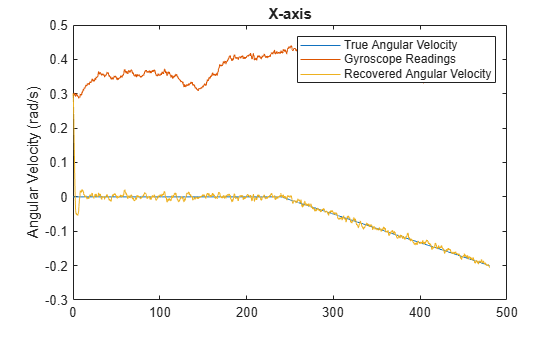```figure(2) plot(time,angVelBody(:,2), ... time,gyroReadingsBody(:,2), ... time,angVelBodyRecovered(:,2)) title('Y-axis') ylabel('Angular Velocity (rad/s)')```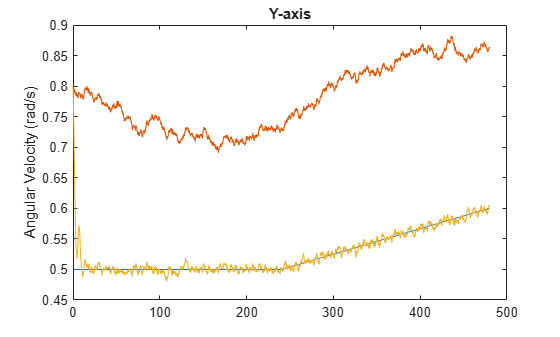```figure(3) plot(time,angVelBody(:,3), ... time,gyroReadingsBody(:,3), ... time,angVelBodyRecovered(:,3)) title('Z-axis') ylabel('Angular Velocity (rad/s)') xlabel('Time (s)')```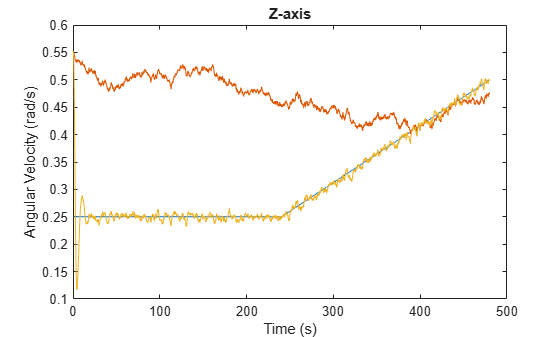## Algorithms

expand all

Note: The following algorithm only applies to an NED reference frame.

The `imufilter` uses the six-axis Kalman filter structure described in . The algorithm attempts to track the errors in orientation, gyroscope offset, and linear acceleration to output the final orientation and angular velocity. Instead of tracking the orientation directly, the indirect Kalman filter models the error process, x, with a recursive update:

`${x}_{k}=\left[\begin{array}{c}{\theta }_{k}\\ {b}_{k}\\ {a}_{k}\end{array}\right]={F}_{k}\left[\begin{array}{c}{\theta }_{k-1}\\ {b}_{k-1}\\ {a}_{k-1}\end{array}\right]+{w}_{k}$`

where xk is a 9-by-1 vector consisting of:

• θk –– 3-by-1 orientation error vector, in degrees, at time k

• bk –– 3-by-1 gyroscope zero angular rate bias vector, in deg/s, at time k

• ak –– 3-by-1 acceleration error vector measured in the sensor frame, in g, at time k

• wk –– 9-by-1 additive noise vector

• Fk –– state transition model

Because xk is defined as the error process, the a priori estimate is always zero, and therefore the state transition model, Fk, is zero. This insight results in the following reduction of the standard Kalman equations:

Standard Kalman equations:

`$\begin{array}{l}{x}_{k}^{-}={F}_{k}{x}_{k-1}^{+}\\ {P}_{k}{}^{-}={F}_{k}{P}_{k-1}^{+}{F}_{k}^{T}+{Q}_{k}\\ \\ {y}_{k}={z}_{k}-{H}_{k}{x}_{k}^{-}\\ {S}_{k}\text{\hspace{0.17em}}={R}_{k}+{H}_{k}{P}_{k}^{-}{H}_{k}{}^{T}\\ {K}_{k}={P}_{k}^{-}{H}_{k}^{T}{\left({S}_{k}\right)}^{-1}\\ {x}_{k}^{+}={x}_{k}^{-}+{K}_{k}{y}_{k}\\ {P}_{k}^{+}={P}_{k}{}^{-}-{K}_{k}{H}_{k}{P}_{k}{}^{-}\end{array}$`

Kalman equations used in this algorithm:

`$\begin{array}{l}{x}_{k}^{-}=0\\ {P}_{k}{}^{-}={Q}_{k}\\ \\ {y}_{k}={z}_{k}\\ {S}_{k}\text{\hspace{0.17em}}={R}_{k}+{H}_{k}{P}_{k}^{-}{H}_{k}{}^{T}\\ {K}_{k}={P}_{k}^{-}{H}_{k}^{T}{\left({S}_{k}\right)}^{-1}\\ {x}_{k}^{+}={K}_{k}{y}_{k}\\ {P}_{k}^{+}={P}_{k}{}^{-}-{K}_{k}{H}_{k}{P}_{k}{}^{-}\end{array}$`

where

• xk –– predicted (a priori) state estimate; the error process

• Pk –– predicted (a priori) estimate covariance

• yk –– innovation

• Sk –– innovation covariance

• Kk –– Kalman gain

• xk+ –– updated (a posteriori) state estimate

• Pk+ –– updated (a posteriori) estimate covariance

k represents the iteration, the superscript + represents an a posteriori estimate, and the superscript represents an a priori estimate.

The graphic and following steps describe a single frame-based iteration through the algorithm.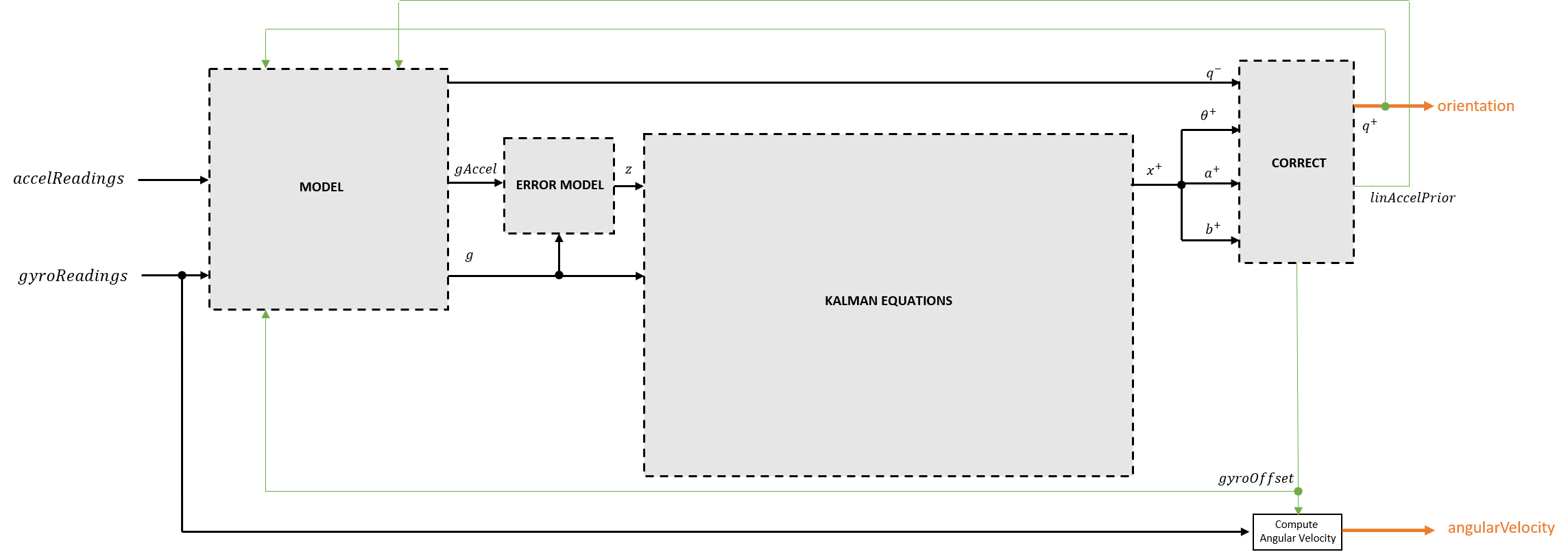Before the first iteration, the `accelReadings` and `gyroReadings` inputs are chunked into 1-by-3 frames and `DecimationFactor`-by-3 frames, respectively. The algorithm uses the most current accelerometer readings corresponding to the chunk of gyroscope readings.

 Roetenberg, D., H.J. Luinge, C.T.M. Baten, and P.H. Veltink. "Compensation of Magnetic Disturbances Improves Inertial and Magnetic Sensing of Human Body Segment Orientation." IEEE Transactions on Neural Systems and Rehabilitation Engineering. Vol. 13. Issue 3, 2005, pp. 395-405.